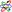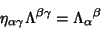## Minkowski Space

A 4-D space with the Minkowski Metric. Alternatively, it can be considered to have a Euclidean Metric, but with its Vectors defined by(1)

whereis the speed of light.The Metric is Diagonal with(2)

so(3)

Letbe the Tensor for a Lorentz Transformation. Then(4)(5)(6)

The Necessary and Sufficient conditions for a metricto be equivalent to the Minkowski metricare that the Riemann Tensor vanishes everywhere () and that at some pointhas three Positive and one Negative Eigenvalues.